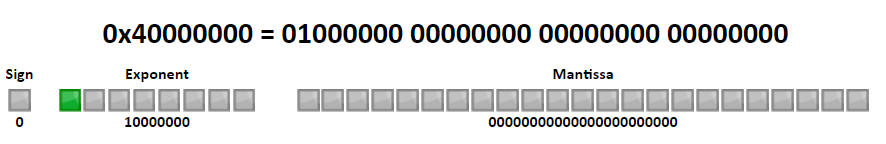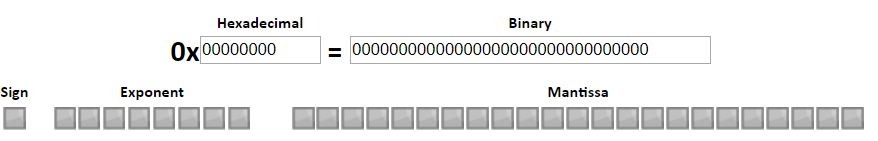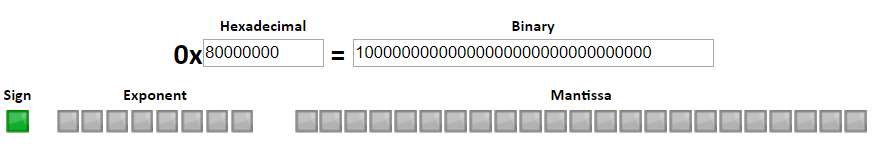Over a million developers have joined DZone.{{announcement.body}}
{{announcement.title}}

# The Issue of Negative Zero

DZone 's Guide to

# The Issue of Negative Zero

### Let's take a look at the issue of negative zero.

· Database Zone ·
Free Resource

Comment (0)

Save
{{ articles.views | formatCount}} Views

We got a bug report recently about RavenDB messing up a query. The query in question was:

from index TestIndex where Amount < 0

The query, for what it is worth, is meant to find accounts that have pre-paid. The problem was that this query returned bad results. This was something very strange. Comparisons of numbers is a very well-trodden field in RavenDB.

How can this be? Let's look a bit deeper into things, shall we? Luckily, the reproduction is pretty simple, let's take a look:

``````from o in docs.Orders
select new {
Amount = -1.5m + 1.5m
}``````

With an index like this, we can be fairly certain that the query above will return no results, right? After all, this isn't any kind of complex math. But the query will return results for this index. And that is strange.

You might have noticed that the numbers we use are decimals ( 1.5m is the C# postfix for decimal constant ). The problem repeats with decimal, double and float. When we started looking at this, we assumed that the problem was with floating point precision. After all, any comparison of floating point values will warn you about this. But using decimal, we are supposed to be protected from this.

When faced with such an issue, we dove deep into how RavenDB does numeric range comparisons. Sadly, this is not a trivial matter, but after reviewing everything, we were certain that the code is correct, it had to be something else.

I finally ended up with the following code:

``````int[] parts = decimal.GetBits(decimal.Zero);

Console.WriteLine(\$"{parts:x8} - {parts:x8} - {parts:x8} - {parts:x8}");

decimal zero = -1.5m + 1.5m;
parts = decimal.GetBits( zero);

Console.WriteLine(\$"{parts:x8} - {parts:x8} - {parts:x8} - {parts:x8}");

Console.WriteLine(\$"{zero} == {decimal.Zero} ? {zero == decimal.Zero}");``````

And that gave me some interesting output:

``````00000000 - 00000000 - 00000000 - 00000000
00000000 - 00000000 - 00000000 - 80010000

0.0 == 0 ? True``````

And that was quite surprising. We can get the same using double of floats:

``````Console.WriteLine(BitConverter.ToString(BitConverter.GetBytes(0.0d)));
Console.WriteLine(BitConverter.ToString(BitConverter.GetBytes(-0.0d)));
Console.WriteLine(BitConverter.ToString(BitConverter.GetBytes(0.0f)));
Console.WriteLine(BitConverter.ToString(BitConverter.GetBytes(-0.0f)));``````

And this code gives us:

``````00-00-00-00-00-00-00-00
00-00-00-00-00-00-00-80
00-00-00-00
00-00-00-80``````

What is going on here?

Well, let's look at the format of floating point numbers for a sec, shall we? There is a great discussion of this here, but the key observation for this particular issue can be seen by looking at the binary representation.

Here is 2.0:And is here -2.0:As usual, the first bit is the sign marker. But unlike int32 or int64, with floating point, it is absolutely legal to have the following byte patterns:The first one here is zero, and the second one is negative zero. They are equal to one another, but the binary representation is different. And that caused our issue.

Part of how RavenDB does range numeric queries on floating point it to translate them to a lexical value that can be compared using memcmp(), there is some magic involved, but the key observation was that we didn't account for negative zero in the problem. The negative zero was translated to -9,223,372,036,854,775,808 (long.MinValue) and that obviously is smaller than zero.

The fix in our code was to handle this explicitly, like so:

``````if (value == 0)
{
// this is not superflous code, we need
// to account for negative zeroes here
value = 0;
}``````

And that is how I started out by chasing bugs and ended up with a sum total of negative zero.

Topics:
database ,negative zero ,tutorial ,ravendb

Comment (0)

Save
{{ articles.views | formatCount}} Views

Published at DZone with permission of

Opinions expressed by DZone contributors are their own.

# {{ parent.title || parent.header.title}}

{{ parent.tldr }}

{{ parent.urlSource.name }}